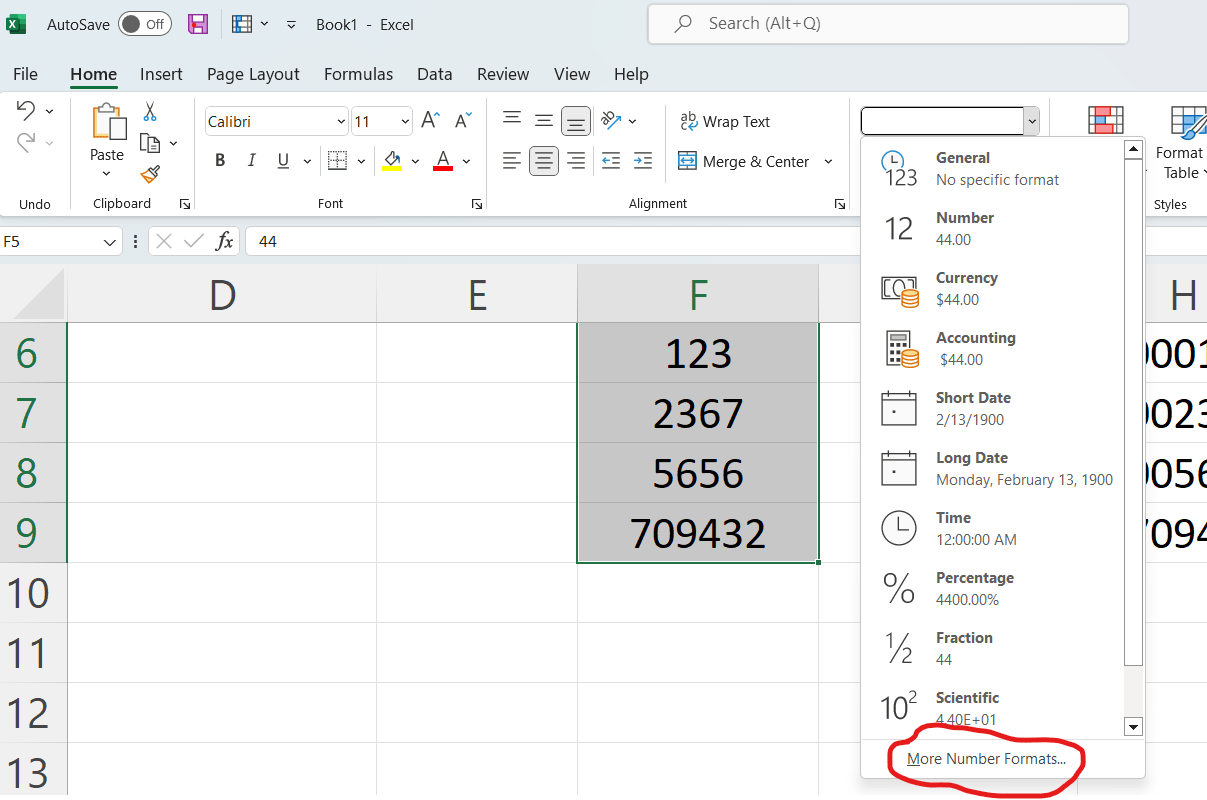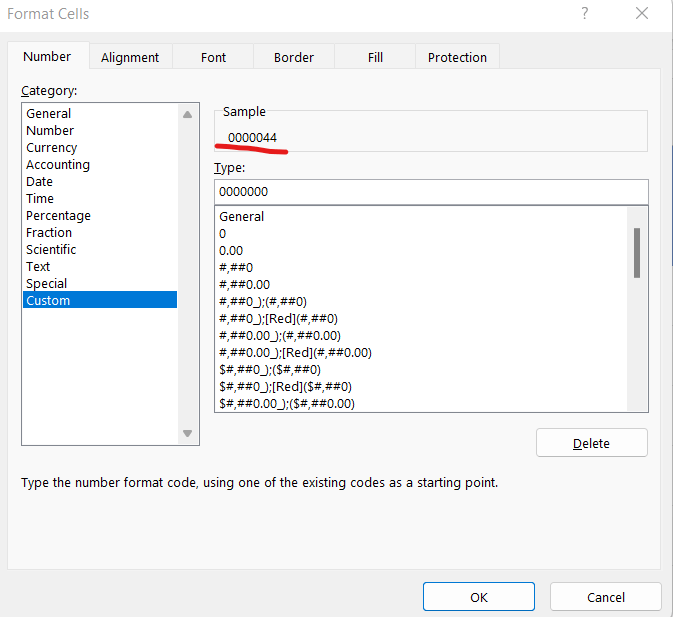1. TEXT Function
2. REPT Function
3. Custom Number Format

The TEXT functions allows you to add to set the number of leading leading zeros by set the length of the text. So in this case we want to ensure that we have a length of seven values. So it will replace any spaces with lack of characters with a zero.

``=TEXT(F2,"0000000")``

This method is only slightly more complex than using the TEXT function. This function will also evaluate the length of the characters in the cell and then apply the number of zeros necessary. However, in this case, you will need to manually specify the length of the cell contents with the LEN function.

``=REPT("0",7-LEN(F2))&F2``

By evaluating this function, you can see that we are replicating the “0” value based on the result of the LEN() function which returns the length of the cell contents. At the end we are adding the back the cell contents.

We can achieve the same result by using the custom format to specify the length of cell contents by using 7 zeros. This would be mean that the cell contents would make up the difference.

1. Highlight the column.
2. Click dropdown in the top ribbon for Number Format section
3. Choose More Number Formats
4. Choose Custom under the Category Sections
5. Add the number of zeros you need to complete the lengthAdding the number zeros as the total length of the cell contents you need will update the value in the preview.• in
• |
• November 11, 2022#### Gaelim Holland

Subscribe
Notify of